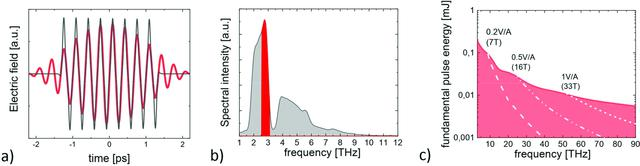disable zoom     view article Figure 2 THz waveforms emitted by the THz undulator for a central frequency of ∼3 THz (a) and corresponding THz spectrum (b). Data are shown for the total emitted THz spectrum (black) and for a bandpass filter of 20% around the fundamental frequency (red). The pulse energies as emitted into a bandwidth of 20% around the fundamental are shown in (c). The waveforms are derived from an analytical calculation [described in detail by Tanikawa et al. (2019)] assuming the most optimal bunch charge and corresponding bunch form (<5.8 THz/500 pC, <15 THz/250 pC, <75 THz/100 pC and 20 pC at higher frequencies). The achievable peak THz fields, assuming a moderate numerical aperture of 0.25, are indicated in (c) by dashed lines. Note that one does not expect to observe significant changes in the electron bunch form for beam energies between 8.5 and 17.5 GeV and hence the THz pulse energies are the same.JOURNAL OFSYNCHROTRONRADIATION
ISSN: 1600-5775
Volume 27| Part 3| May 2020| Pages 796-798